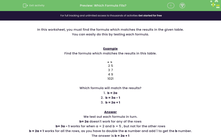# Find the Correct Formula

In this worksheet, students will find the formula that matches the data in the given table.Key stage:  KS 3

Curriculum topic:   Algebra

Curriculum subtopic:   Use Standard Mathematical Formulae

Popular topics:   Algebra worksheets, Year 7 Algebra worksheets

Difficulty level:#### Worksheet Overview

In this activity, we will be finding the formula that matches the results in the given table.

Example

Find the formula that matches the results in this table.

a b
2 5
3 7
4 9
10 21

Which formula will match the results?

1.  b = 2a

2.  b = 3a - 1

3.  b = 2a + 1

We test out each formula in turn.

b = 2a doesn't work for any of the rows.

b = 3a - 1 works for when a = 2 and b = 5 , but not for the other rows.

b = 2a + 1 works for all the rows, as you have to double the a number and add 1 to get the b number.

So, the answer is b = 2a + 1

Shall we have a go at this now in the following questions?### What is EdPlace?

We're your National Curriculum aligned online education content provider helping each child succeed in English, maths and science from year 1 to GCSE. With an EdPlace account you’ll be able to track and measure progress, helping each child achieve their best. We build confidence and attainment by personalising each child’s learning at a level that suits them.

Get started